Test: Laplace Transform- 1

# Test: Laplace Transform- 1

Test Description

## 10 Questions MCQ Test GATE ECE (Electronics) 2023 Mock Test Series | Test: Laplace Transform- 1

Test: Laplace Transform- 1 for Electronics and Communication Engineering (ECE) 2023 is part of GATE ECE (Electronics) 2023 Mock Test Series preparation. The Test: Laplace Transform- 1 questions and answers have been prepared according to the Electronics and Communication Engineering (ECE) exam syllabus.The Test: Laplace Transform- 1 MCQs are made for Electronics and Communication Engineering (ECE) 2023 Exam. Find important definitions, questions, notes, meanings, examples, exercises, MCQs and online tests for Test: Laplace Transform- 1 below.
Solutions of Test: Laplace Transform- 1 questions in English are available as part of our GATE ECE (Electronics) 2023 Mock Test Series for Electronics and Communication Engineering (ECE) & Test: Laplace Transform- 1 solutions in Hindi for GATE ECE (Electronics) 2023 Mock Test Series course. Download more important topics, notes, lectures and mock test series for Electronics and Communication Engineering (ECE) Exam by signing up for free. Attempt Test: Laplace Transform- 1 | 10 questions in 30 minutes | Mock test for Electronics and Communication Engineering (ECE) preparation | Free important questions MCQ to study GATE ECE (Electronics) 2023 Mock Test Series for Electronics and Communication Engineering (ECE) Exam | Download free PDF with solutions
 1 Crore+ students have signed up on EduRev. Have you?
Test: Laplace Transform- 1 - Question 1

### A voltage having the laplace transform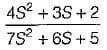is applied across a 2H inductor having zero initial current What is the current in the inductor at t = ∞?

Test: Laplace Transform- 1 - Question 2

### The function f (t) shown in figure will have laplace transform as: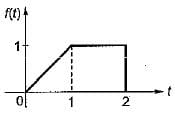Test: Laplace Transform- 1 - Question 3

### Which of the following is the correct Laplace transform of the signal in the given figure?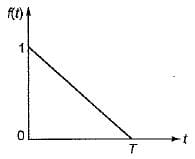Test: Laplace Transform- 1 - Question 4

Given: x(t) =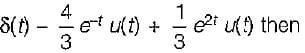find x(s) and its ROC:

Test: Laplace Transform- 1 - Question 5

Given: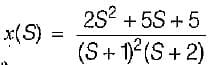and Re [S] >-1 then x (t):

Test: Laplace Transform- 1 - Question 6

If the input to LTI system is x(t) = e-3t u(t). Then the output is y(t) = [e-t - e-2t] u(t) the transfer function of the system is

Detailed Solution for Test: Laplace Transform- 1 - Question 6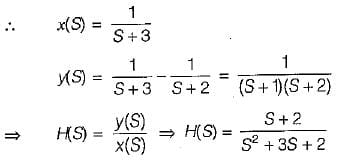Test: Laplace Transform- 1 - Question 7

If,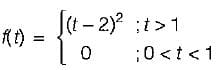then the value of L{tf(t)} is equal to

Test: Laplace Transform- 1 - Question 8

The transfer functions of the sytem is given by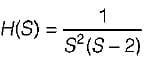The impulse response of the system is

Detailed Solution for Test: Laplace Transform- 1 - Question 8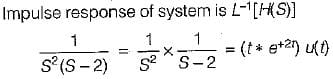Test: Laplace Transform- 1 - Question 9

The Laplace transform of a continuous signal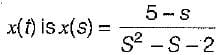If the Fourier transform of the signal exists, then x(t) is

Detailed Solution for Test: Laplace Transform- 1 - Question 9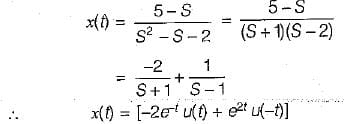Test: Laplace Transform- 1 - Question 10

The laplace transform of i(t) is given by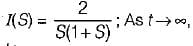the value of i(t) tends to

Detailed Solution for Test: Laplace Transform- 1 - Question 10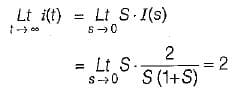## GATE ECE (Electronics) 2023 Mock Test Series

21 docs|263 tests
Information about Test: Laplace Transform- 1 Page
In this test you can find the Exam questions for Test: Laplace Transform- 1 solved & explained in the simplest way possible. Besides giving Questions and answers for Test: Laplace Transform- 1, EduRev gives you an ample number of Online tests for practice

## GATE ECE (Electronics) 2023 Mock Test Series

21 docs|263 tests(Scan QR code)